Next: A Gravitational Wave Map Previous: Critical Frequencies and Gravitational

Ā

## Amplitude Distribution

In this section we consider the GWB spectrum as such, that is, we study how dimensionless strain metric amplitude h is dependent on frequency. For a sample of N independent binaries emitting GW at a given frequency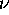the net result is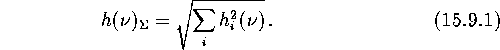If one uses the frequency interval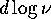, the result will be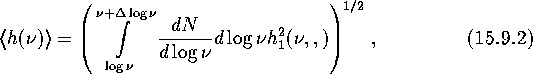where

Āis the familiar amplitude produced by an individual binary,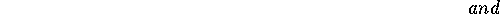are, respectively, effective distance to the sample and effective mass of the binaries (in solar units) producing the GW radiation at the frequency. For example, for a homogeneous sample (which is a good approximation to extragalactic binaries)Ā one obtains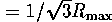, where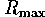is the outer boundary of the sample.

Making use of expressions (15.3.2), (15.3.3) and (15.9.3) yields

Ā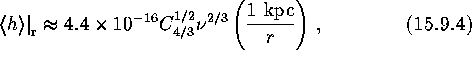for the red end of the spectrum () and

Ā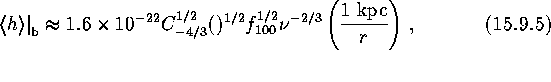for the blue end.

One may be interested in how the GWB from the external object relates to that from our Galaxy, providing that the observations are being performed with a detector of angular resolution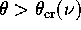such that the Galaxy is still not transparent at the frequency of observations, and the object is inside the detector's beam,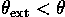. In this case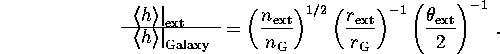Here n is the number of stars within the object,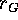is an effective ``radius'' of the Galaxykpc. Some specific examples are shown in TableĀ13.

Ā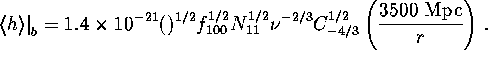It can be seen from TableĀthat only the closest stellar systems (such as Magellanic CloudsĀ and M31) can noticeably affect the galactic binary background. The level of the cosmological binary GWB is always an order of magnitude below the galactic level. Indeed, in analogy with expressions (15.9.4) and (15.9.5) one obtains for the red (flat) and blue (decreasing) parts of the spectrum, respectively: \$\$ .h|_r =2.1 10^-17 n_11^1/2N_11^1/2^2/3 C_4/3^1/2(3500 Mpcr),

Their comparison with expressions (15.9.4) and (15.9.5) yieldsfor both ends of the spectrum.

The GW noise produced by extragalactic binary systemsĀ is still considerably higher than the expected amplitudes of relic gravitational waves produced in well-known inflationary models (Rubakov et al., 1982):, even if one conducts observations at the galactic poles which are poor in stars.

ĀFigure 43: Distribution of galaxies from Tully's Catalog by distance.ĀNext: A Gravitational Wave Map Previous: Critical Frequencies and Gravitational

Mike E. Prokhorov
Sat Feb 22 18:38:13 MSK 1997